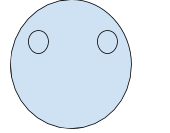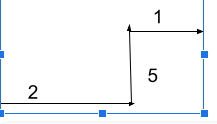0
738

# Logical Reasoning Questions for CMAT & TMAT PDF

Download CMAT and TISSNET Logical Reasoning Questions and Answers PDF covering the important questions. Most expected LR questions with explanations for CMAT & TISSNET 2021 exams.

Question 1: In the following question, + means -; – means x ; x means ➗; ➗ means +
Then find the value of 16+4-3➗22×11

a) 6

b) 15

c) 19

d) 18

Question 2: Which letter is 5th to the right of the 7th letter from the left end?
AXMBHLYUPWERHDGFT

a) R

b) H

c) E

d) W

Question 3: Identify the odd man out

a) Potato

b) Carrot

c) Ginger

d) Brinjal

Question 4: Choose the right answer, based on the relationship given in question.
Milk:White

a) Book:Pages

b) Bag:Book

c) Blood:Red

d)  Sky: Dark

Question 5: MANASA is coded as OCPCUC then how will RAJINI be coded as ?

a) TDLKMJ

b) TDLNKP

c) TDPLMO

d) TCLKPK

Question 6: What comes next ?
1,5,14,30,?

a) 85

b) 55

c) 78

d) 22

Question 7: How is this represented using the Venn diagram?
Classroom,Student,Board

a)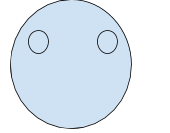b)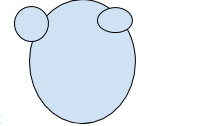c)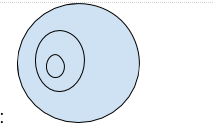d)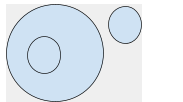Question 8: Raju was standing at a panipuri stall facing to the north. His friend Rani called him to tell her house address. She told him to turn right and walk 2 km where he can find a bookstall. From there she told him to take left and walk 5 km where he can find a sweet shop. Then from there she asked him to take a right turn and walk 1km to come to her home. In what direction is Raju from his starting position?

a) North

b) South

c) North-east

d) North west

e) e

From the given data, the question can be written as
16-4×3+22➗11
=&amp;gt; according to BODMAS, 22/11=2;
-4×3=-12
=&amp;gt;16-12+2
=6

5th to the right of 7th letter from left end is 12th letter from left end
i.e; R is the 12th letter

All the vegetables except Brinjal are grown inside the land

The question relation is telling that milk is in white colour,
If we look at the options, option c is best suited relation similar to the question relation
i.e; Blood is in red colour

From the given question MANASA has become OCPCUC
The logic behind this is M+2=O
A+2=C
N+2=P
A+2=C
S+2=U
A+2=C
Similarly, for RAJINI, R+2=T
A+2=C
J+2=L
I+2=K
N+2=P
I+2= K

the series is 1, $2^{2}$ +1 = 1,5
Similarly, 30+square of 5 will be the answer
30+25=55